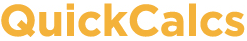1. Select category 2. Choose calculator 3. Enter data 4. View results

## Interpret P values

### Compute post test probability

To interpret a "statistically significant" P value, you need to take into account the context of the experiment, as expressed by the prior probability that your hypothesis is true. Read more.
 Alpha: Power (%): Prior probability (%)

### Multiple comparisons correction

It is easy to interpret a single P value. It is the chance of obtaining a difference (correlation, association, etc.) as striking as what you observed (or more so) by chance if there really is no difference (no correlation, no association, etc.). It is harder to interpret a small P value when you compute many. Clearly, the chances of observing a small P value by coincidence of random sampling is higher when you make many comparisons. Read more about multiple comparisons.

Enter your P value, and the number of independent comparisons you made, and this calculator will interpret your result.

P value:
Number of independent comparisons: